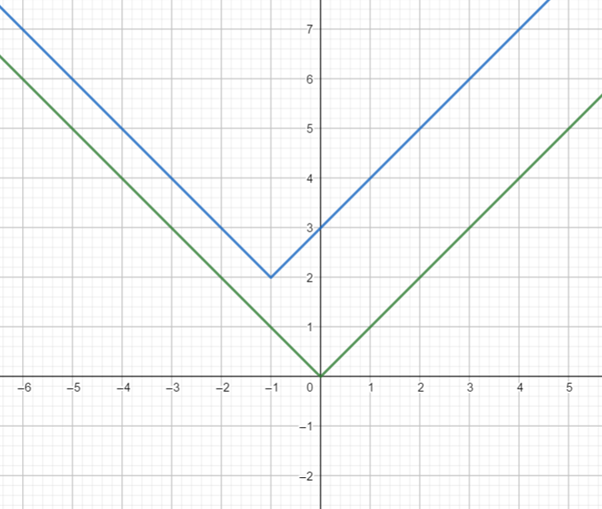# the formula of function g .### Precalculus: Mathematics for Calcu...

6th Edition
Stewart + 5 others
Publisher: Cengage Learning
ISBN: 9780840068071### Precalculus: Mathematics for Calcu...

6th Edition
Stewart + 5 others
Publisher: Cengage Learning
ISBN: 9780840068071

#### Solutions

Chapter 2.5, Problem 57E
To determine

## To find : the formula of function g .

Expert Solution

The function is g=|x+1|+2 .

### Explanation of Solution

Given information : the function is f=|x|Form the above graph it is observed that the graph of the function of g is the graph of f=|x| shifted 1 unit left and shift 2 unit upward.

Use the definition of horizontal and vertically shifts of graph,

Then for the function of gsubstitute x by (x+1) then add 2 in the function f=|x| .

Hence, the function g=|x+1|+2 .

### Have a homework question?

Subscribe to bartleby learn! Ask subject matter experts 30 homework questions each month. Plus, you’ll have access to millions of step-by-step textbook answers!